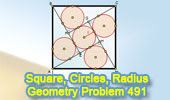## Wednesday, August 4, 2010

### Problem 491: Square, Right Triangle, Incircle, Inscribed Circle, Radius

Geometry Problem
Click the figure below to see the complete problem 491 about Square, Right Triangle, Incircle, Inscribed Circle, Radius.See also:
Complete Problem 491

Level: High School, SAT Prep, College geometry

#### 5 comments:

1.In ▲AHD
1) x = (a+b-c)/2 => AE = a/2 - x
2) from pythagore theorem
x = (-a + a√3)/4

2.Let the tangent from A to the nearest circle=z. We can now write the following equations immediately: 2z + 2r = a -----(1) and a^2=(z+3r)^2+(z+r)^2 -----(2) from which z can be eliminated to obtain: r=a(√3-1)/4
Ajit

3.I'm missing something.... how do you get the 2z + 2r = a equation from?

4.In any right angled triangle such as ABE, we've: AE+BE-AB=2*(In-radius) and hence in our notation: (z+r)+(z+3r)-a=2r or a=2z+2r.
Ajit

1.How was BE found to be z+3r?